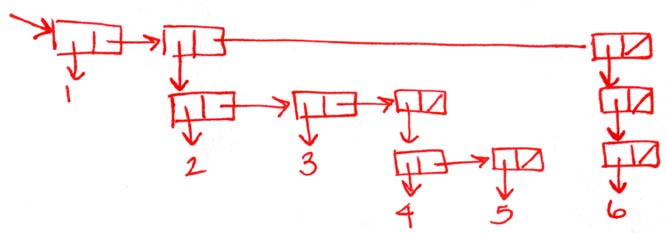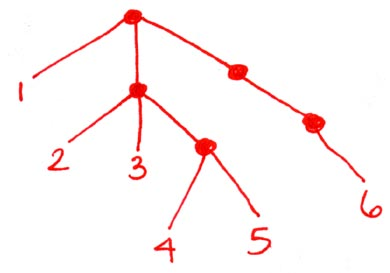```recitation 7: more practice with higher-order procedures

--------------------------------------------
(define (map proc lst)
(if (null? lst) nil
(cons (proc (car lst))
(map proc (cdr lst)))))

(define (filter pred lst)
(cond ((null? lst) nil)
((pred (car lst))
(cons (car lst) (filter pred (cdr lst))))
(else (filter pred (cdr lst)))))

(define (fold-right op init lst)
(if (null? lst)
init
(op (car lst)
(fold-right op init (cdr lst)))))

--------------------------------------------
Suppose lst is bound to the list (1 2 3 4 5 6 7). Using map, filter,
and/or fold-right, write an expression involving lst that returns:

(1 4 9 16 25 36 49)
(map square lst)

(1 3 5 7)
(filter odd? lst)

((1 1) (2 2) (3 3) (4 4) (5 5) (6 6) (7 7))
(map (lambda (x) (list x x)) lst)

((2) ((4) ((6) \#f)))
(fold-right list nil (map list (filter even? lst)))

The maximum element of lst: 7
(fold-right max (car lst) lst)

The last pair of lst: (7)
This is a trick question.  You can't get a hold of the last pair of
the input list since map, filter & fold-right strip away the top level
list and just hand you the contents.

--------------------------------------------
(intersection (list 1 2 3 4) (list 3 5 1 6)) => (1 3)

* type of intersection: List<A>,List<A> -> List<A>

* implement intersection using map, filter, and/or fold-right

(define (intersection a b)
(filter (lambda (x) (member x b)) a))

--------------------------------------------
(apply-all (list abs square cube inc) -4) => (4 16 -64 -3)

* type of apply-all: List<A->B>,A -> List<B>

* implement apply-all recursively

(define (apply-all ops arg)
(if (null? ops)
nil
(cons ((car ops) arg)
(apply-all (cdr ops) arg))))

* implement apply-all using map, filter and/or fold-right

(define (apply-all ops x)
(map (lambda (op) (op x)) ops))

--------------------------------------------
(append (list 1 2 3) (list 4 5 6)) => (1 2 3 4 5 6)

* type of append: List<A>,List<A> -> List<A>

* implement append recursively

(define (append a b)
(if (null? a) b
(cons (car a) (append (cdr a) b))))

* implement append using map, filter and/or fold-right

(define (append a b)
(fold-right cons b a))

--------------------------------------------
(reverse (list 1 2 3 4)) => (4 3 2 1)

* type of reverse:List<A> -> List<A>

* implement reverse recursively using append

(define (reverse lst)
(if (null? lst)
nil
(append (reverse (cdr lst))
(list (car lst)))))

* implement reverse iteratively

(define (reverse lst)
(if (null? lst)
(reverse-helper (cdr lst)
(reverse-helper lst nil))

---------------------------------------------------------------------------------------
(define my-tree (list 1 (list 2 3 (list 4 5)) (list (list 6))))

* draw the box & pointer diagram for my-tree* how does my-tree print?
(1 (2 3 (4 5)) ((6)))

* draw a "stylized tree diagram" for my-tree* how are they related?
Each node of the tree is a proper list in the box & pointer diagram.
If the list has n elements, the node has n branches.

--------------------------------------------
(define (leaf? tree) (not (pair? tree)))

(define (tree-manip leaf-op init merge tree)
(if (null? tree)
init
(if (leaf? tree)
(leaf-op tree)
(merge (tree-manip leaf-op init merge (car tree))
(tree-manip leaf-op init merge (cdr tree))))))

* type of tree-manip: A->B, B, (B,B->B), tree<A> -> B

* use tree manip to square every element of a tree

(square-tree my-tree)  =>  (1 (4 9 (16 25)) ((36)))

(define (square-tree tree)
(tree-manip square nil cons tree))

* use tree-manip to compute the product of all the even-valued
leaves of the tree

(product-even my-tree)   =>  48

(define (product-even tree)
(tree-manip (lambda (x) (if (odd? x) 1 x)) 1 * tree))

* use tree-manip flatten a tree

(flatten my-tree)   =>   (1 2 3 4 5 6)

(define (flatten tree)
(tree-manip (lambda (x) (list x)) nil append tree))

* use tree-manip to "deep-reverse" a tree (reverse the order of
children at each node)

(deep-reverse my-tree)  =>  (((6)) ((5 4) 3 2) 1)

(define (deep-reverse tree)
(tree-manip
(lambda (x) x)
nil
(lambda (x y) (append y (list x)))
tree))

* use tree-manip to sum up the values of the leaves of the tree

(sum-leaves my-tree)  =>  21

(define (sum-leaves tree)
(tree-manip (lambda (x) x) 0 + tree))

* use tree-manip to create a new tree, which keeps the odd-valued
leaves of the original tree within the same tree structure, but
removes even valued leaves

(keep-odd-leaves my-tree) =>  (1 (3 (5)))
(keep-odd-leaves (list 1 2 3 4 5 6))  =>  (1 3 5)
(keep-odd-leaves (list 2 4 6))  =>  nil
(keep-odd-leaves (list (list 1) (list 4) (list 6))) =>  ((1))

(define (keep-odd-leaves tree)
(tree-manip
(lambda (x) (if (even? x) nil x))
nil
(lambda (x y) (if (null? x) y (cons x y)))
tree))

* for each of the above exercises, write down the types of arguments &
return value for the calls to tree-manip

```